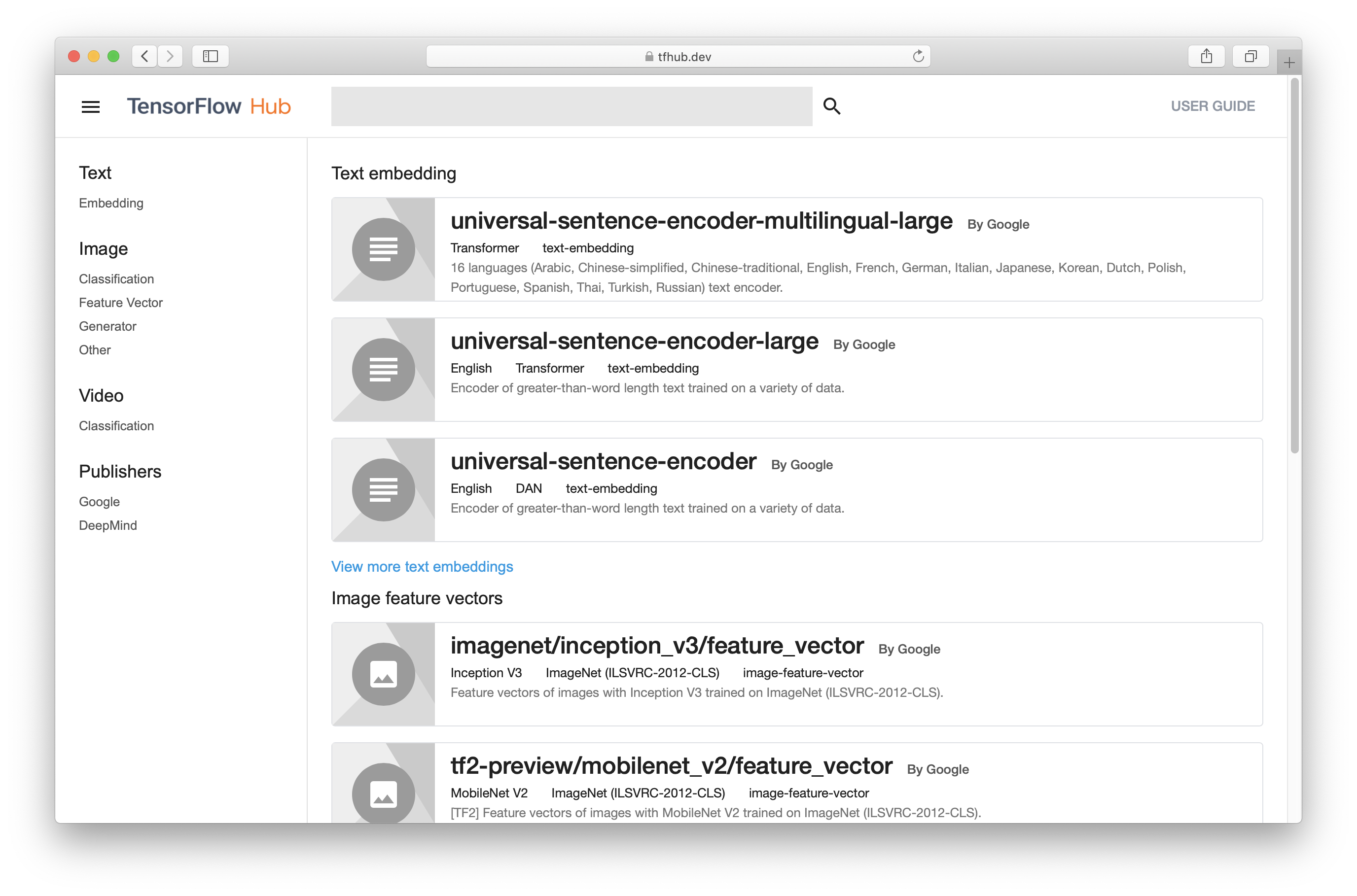# Overview In tfhub: Interface to 'TensorFlow' Hub

The tfhub package provides R wrappers to TensorFlow Hub.

TensorFlow Hub is a library for reusable machine learning modules.

TensorFlow Hub is a library for the publication, discovery, and consumption of reusable parts of machine learning models. A module is a self-contained piece of a TensorFlow graph, along with its weights and assets, that can be reused across different tasks in a process known as transfer learning. Transfer learning can:

• Train a model with a smaller dataset,
• Improve generalization, and
• Speed up training.

## Installation

You can install the released version of tfhub from CRAN with:

```install.packages("tfhub")
```

And the development version from GitHub with:

```# install.packages("devtools")
devtools::install_github("rstudio/tfhub")
```

After installing the tfhub package you need to install the TensorFlow Hub python module:

```library(tfhub)
install_tfhub()
```

Modules can be loaded from URL's and local paths using `hub_load()`

```module <- hub_load("https://tfhub.dev/google/tf2-preview/mobilenet_v2/feature_vector/2")
```

Module's behave like functions and can be called with Tensors eg:

```input <- tf\$random\$uniform(shape = shape(1,224,224,3), minval = 0, maxval = 1)
output <- module(input)
```

## Using with Keras

The easiest way to get started with tfhub is using `layer_hub`. A Keras layer that loads a TensorFlow Hub module and prepares it for using with your model.

```library(tfhub)
library(keras)

input <- layer_input(shape = c(32, 32, 3))

output <- input %>%
# we are using a pre-trained MobileNet model!
layer_dense(units = 10, activation = "softmax")

model <- keras_model(input, output)

model %>%
compile(
loss = "sparse_categorical_crossentropy",
metrics = "accuracy"
)
```

We can then fit our model in the CIFAR10 dataset:

```cifar <- dataset_cifar10()
cifar\$train\$x <- tf\$image\$resize(cifar\$train\$x/255, size = shape(224,224))

model %>%
fit(
x = cifar\$train\$x,
y = cifar\$train\$y,
validation_split = 0.2,
batch_size = 128
)
```

## Using with tfdatasets

tfhub can also be used with tfdatasets:

• `hub_text_embedding_column()`
• `hub_sparse_text_embedding_column()`
• `hub_image_embedding_column()`

## Using with `recipes`

tfhub adds a `step_pretrained_text_embedding` that can be used with the recipes package.

An example can be found here.

## tfhub.dev

tfhub.dev is a gallery of pre-trained model ready to be used with TensorFlow Hub.## Try the tfhub package in your browser

Any scripts or data that you put into this service are public.

tfhub documentation built on Dec. 19, 2021, 9:07 a.m.